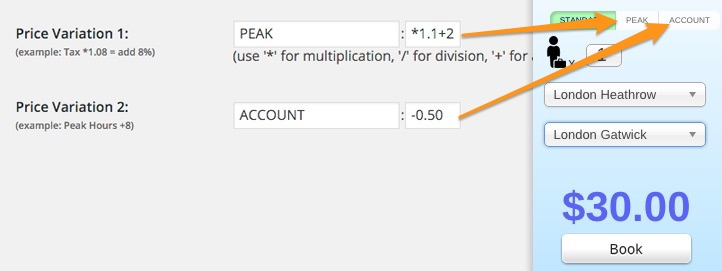In our taxi fare price calculator, Cab Grid Pro there is the ability to display up to two alternative prices. Each price variant is calculated using the standard fare price looked up from your main pricing grid.

When using the price variation options, you would normally add description/instructions to the surrounding content so your customer knows how to use them and/or what they refer to.

If you do not add any formula to the price variation options, no button will be displayed on your plugin. Likewise, if you only want to offer a single price variation, adding only one formula and leaving the other blank will result in only one button showing.

NOTE: This article also applies (in part) to the DISCOUNT CODE feature introduced in Cab Grid Pro v4.0.

Each of the two price variants operates independently on the standard price. They do not effect one another.

## Labels

The first part of the price variation options is a label. This label is displayed on the button shown at the top right of the plugin. Examples of price variation labels you might use include:

• Off-peak
• Night
• Weekend
• Account
• 23:00-06:00
• Discount
• Student
• Holiday

The chosen price variation label is sent with the booking email.

The second box in each price variation option is the formula box. This expects a mathematical formula to be applied to the standard price. The standard price is the price looked up from your pricing table.

### Operators

Symbol Function Examples

# *

Multiply(x): multiply the standard price by the following number. eg. *2 would multiply the standard price by 2 £10 *2 = £20

# /

Divide(÷): divide the standard price by the following number. eg. /2 would divide the standard price by 2 £10 /2 = £5

# +

Add(+): add the following number to the standard price. eg. +2 would add 2 to the standard price £10 +2 = £12

# –

Subtract(-): subtract the following number from the standard price. eg. -2 would subtract 2 from the standard price £10 -2 = £8

### Number Syntax

To indicate a fractional/decimal value in formulae, the decimal point ‘.’ (aka period or full stop) character should be used. For example, the formula for a 5% discount would be *0.95 as opposed to any other notation syntax such as a comma. *0,95 is not valid syntax and will cause an error.

### Percentages

To offer discounts or increase a price based on a percentage you would use the multiplication function.

The formula to increase the price by 10% would be *1.1. This is calculated by dividing the desired percentage by 100 and adding 1. So

#### 10 ÷100 = 0.1 +1 = 1.1

Likewise, to discount a price by 20% would be *0.8. This is calculated by dividing the desired percentage by 100 and subtracting is from 1. So

### Flat rate increases/discounts

You might want to offer a discount or increase based on a flat rate – a standing charge, for example. To do this you would simply use the add (+) operator.

Let’s say you want to add a flat £2 to every journey at peak times. To do this, you would enter the formula:

#### +2

Likewise, to discount by a flat £0.50 you would enter the formula as:

### Combining formula for one price variation

You can enter more complex formula (up to 10 characters long) in each price variation box. This means you can combine operators to further vary prices. For example:

#### *1.25+2.25

This would take the standard price, let’s say £10, add 25% to give £12.50, and then add £2.25, to give £14.75:

#### 10 *1.25 = 12.50 12.50 +2.25 = 14.75

So, you can see you can offer some quite complex price variations at the click of a button. We suggest you try some out formulae to see how they work on your prices.

### Discount/Promotional Codes

Multiple discount codes (promotional codes) can be created and distributed to customers with marketing material. The discount itself (money off) can be entered just like a price variation formula (as detailed above). However, the discount code is entered instead of a label.

The formula is directly applied to the final journey price (before any extras are added), rather than being subtracted…

For example, to offer a 15% discount, the formula entered should be *0.85 – If n is the percentage discount, use this formula to work out the discount:

#### 1-(n÷100)

So 1-(15÷100) = 0.85, the formula for a 15% discount would be *0.85

# Price Variation Options Video Tutorial

x
•
• Discount/Promo Code
• Extras